Next: Exercises Up: Wave Mechanics Previous: Particle in Finite Square

# Square Potential Barrier

Consider a particle of mass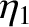and energy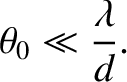interacting with the simple, one-dimensional, potential barrier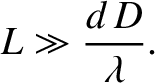(1197)

where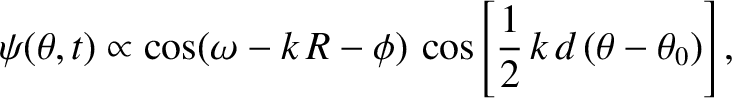. In the regions to the left and to the right of the barrier, the stationary wavefunction,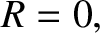, satisfies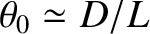(1198)

where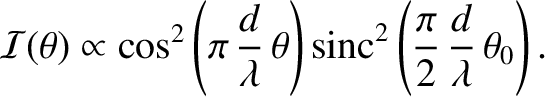(1199)

Let us adopt the following solution of the previous equation to the left of the barrier (i.e.,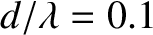):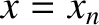(1200)

This solution consists of a plane wave of unit amplitude traveling to the right [because the full wavefunction is multiplied by a factor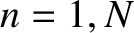)], and a plane wave of complex amplitude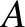traveling to the left. We interpret the first plane wave as an incident particle, and the second as a particle reflected by the potential barrier. Hence,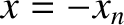is the probability of reflection. (See Sections 7.6 and 12.6.)

Let us adopt the following solution to Equation (1198) to the right of the barrier (i.e.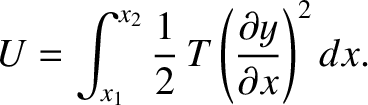):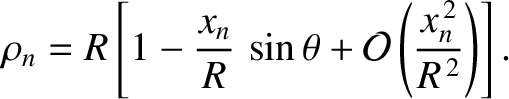(1201)

This solution consists of a plane wave of complex amplitudetraveling to the right. We interpret this as a particle transmitted through the barrier. Hence,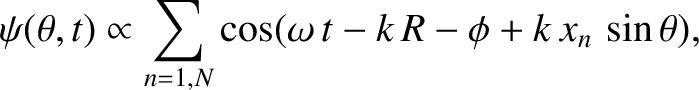is the probability of transmission.

Let us consider the situation in which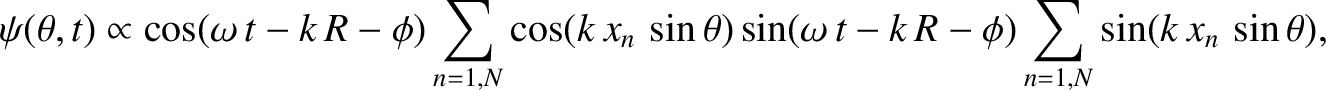. In this case, according to classical mechanics, the particle is unable to penetrate the barrier, so the coefficient of reflection is unity, and the coefficient of transmission zero. However, this is not necessarily the case in wave mechanics. In fact, inside the barrier (i.e.,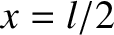),satisfies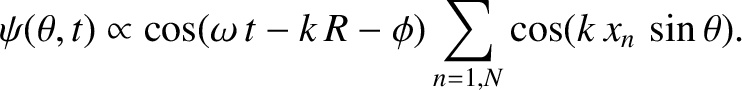(1202)

where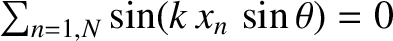(1203)

The general solution to Equation (1202) takes the form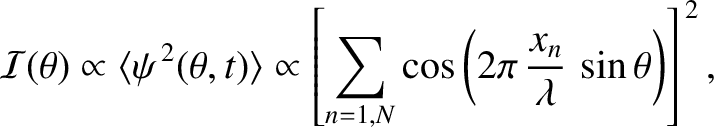(1204)

Continuity of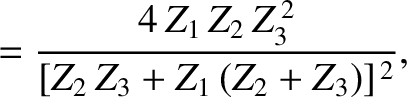and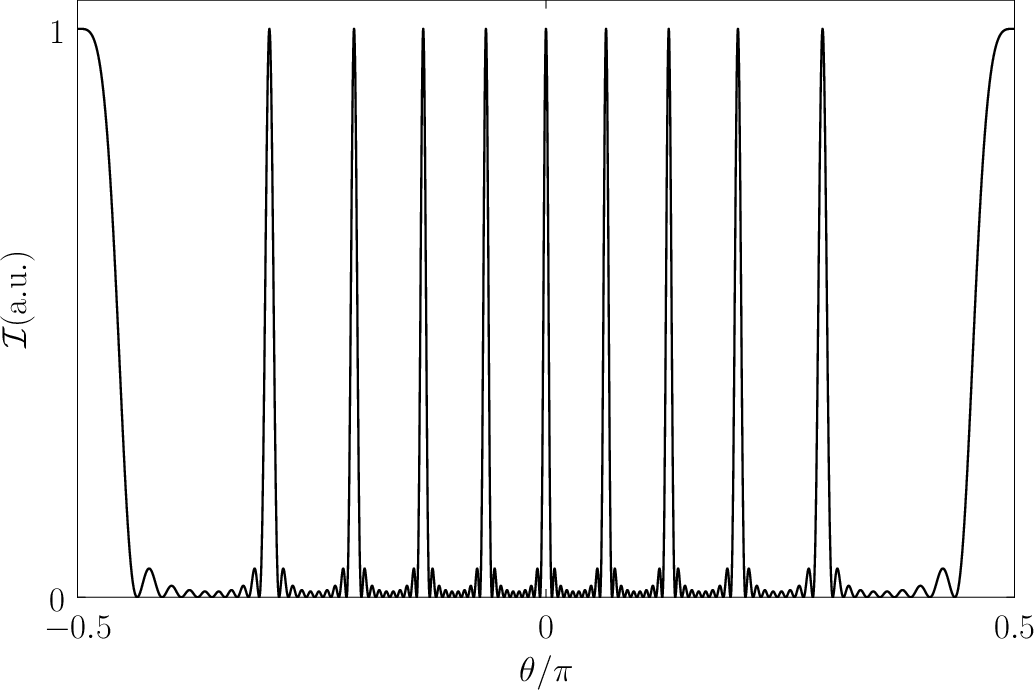at the left edge of the barrier (i.e.,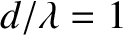) yields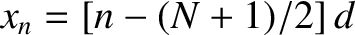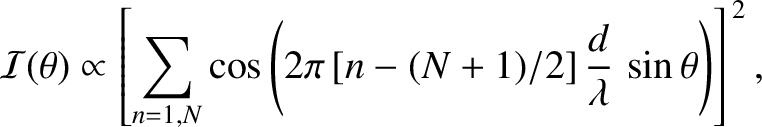(1205)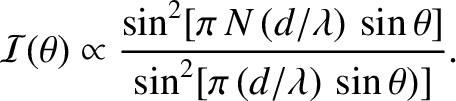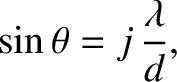(1206)

Likewise, continuity ofandat the right edge of the barrier (i.e.,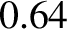) gives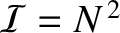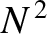(1207)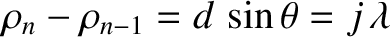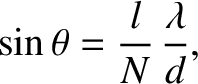(1208)

After considerable algebra (see Exercise 14), the preceding four equations yield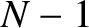(1209)

The fact that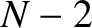ensures that the probabilities of reflection and transmission sum to unity, as must be the case, because reflection and transmission are the only possible outcomes for a particle incident on the barrier. According to Equation (1209), the probability of transmission is not necessarily zero. This means that, in wave mechanics, there is a finite probability for a particle incident on a potential barrier, of finite width, to penetrate through the barrier, and reach the other side, even when the barrier is sufficiently high to completely reflect the particle according to the laws of classical mechanics. This strange phenomenon is known as tunneling. For the case of a very high barrier, such that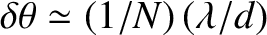, the tunneling probability reduces to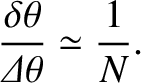(1210)

where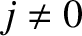is the de Broglie wavelength inside the barrier. Here, it is assumed that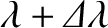. Even in the limit that the barrier is very high, there is an exponentially small, but nevertheless non-zero, tunneling probability. Quantum mechanical tunneling plays an important role in the physics of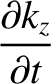-decay and electron field emission (Park 1974).Next: Exercises Up: Wave Mechanics Previous: Particle in Finite Square
Richard Fitzpatrick 2013-04-08